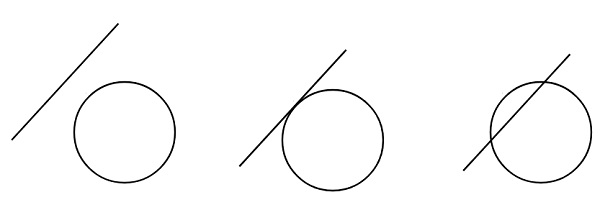# Check if a line touches or intersects a circle in C++

Suppose we have a circle and another straight line. Our task is to find if the line touches the circle or intersects it, otherwise, it passes through outside. So there are three different cases like below −Here we will solve it by following steps. These are like below −

• Find perpendicular P between the center and given a line
• Compare P with radius r −
• if P > r, then outside
• if P = r, then touches
• otherwise inside

To get the perpendicular distance, we have to use this formula (a center point is (h, k))

$$\frac{ah+bk+c}{\sqrt{a^2+b^2}}$$

## Example

Live Demo

#include <iostream>
#include <cmath>
using namespace std;
void isTouchOrIntersect(int a, int b, int c, int h, int k, int radius) {
int dist = (abs(a * h + b * k + c)) / sqrt(a * a + b * b);
cout << "Touching the circle" << endl;
cout << "Intersecting the circle" << endl;
else
cout << "Outside the circle" << endl;
}
int main() {
}
Touching the circle